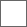### 国产H.265(HEVC)解码器试用报告

(待续)http://xhevc.com/cn/imageCompression/imageCompression.jspx264(x264 - core 129 r2230 1cffe9f - H.264/MPEG-4 AVC codec - Copyleft 2003-2012 - http://www.videolan.org/x264.html - options: cabac=1 ref=9 deblock=1:0:0 analyse=0x3:0x133 me=umh subme=9 psy=0 mixed_ref=1 me_range=24 chroma_me=1 trellis=2 8x8dct=1 cqm=0 deadzone=21,11 fast_pskip=0 chroma_qp_offset=0 threads=6 lookahead_threads=1 sliced_threads=0 nr=0 decimate=1 interlaced=0 bluray_compat=0 constrained_intra=0 bframes=16 b_pyramid=2 b_adapt=2 b_bias=0 direct=3 weightb=1 open_gop=0 weightp=2 keyint=240 keyint_min=23 scenecut=40 intra_refresh=0 rc_lookahead=60 rc=abr mbtree=1 bitrate=250 ratetol=1.0 qcomp=0.60 qpmin=0 qpmax=69 qpstep=4 ip_ratio=1.40 aq=1:0.00):HEVC: 27秒
H264: 19秒d大可以等评测写完之后直接给cnBeta投稿，看看有没有喜闻乐见的喷子们出现